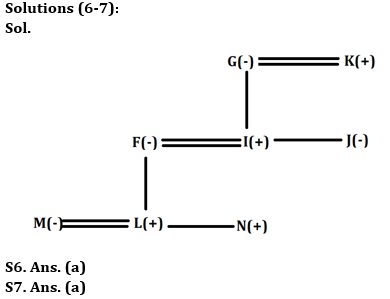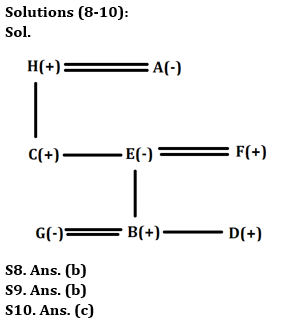Latest Banking jobs   »

# Reasoning Ability Quiz For Bank Foundation 2023 -24th May

Directions (1-2): Answer the questions based on the information given below.
In a family, there are seven kids K, L, M, N, O, P and Q of different heights. Q is shorter than only O. L is just shorter than K. At least three kids are shorter than K. M is taller than P. M is shorter than K. N is not taller than P.

Q1. How many kids are taller than M?
(a) Two
(b) Three
(c) Five
(d) Four
(e) None of these

Q2. Who among the following is third tallest kid?
(a) M
(b) Q
(c) K
(d) L
(e) None of these

Directions (3-5): Answer the questions based on the information given below.
9 trains P, Q, R, S, T, U, V, W and X has different speed. S is faster and slower than at least 3 trains. Four trains are faster and four trains are slower than V. Q is faster than X and P, but slower than S. T is slower than only W. Speed of two trains are in between speed of S and X. R which is faster than at least two trains, is slower than U.

Q3. Which among the following train is slowest train?
(a) P
(b) Q
(c) X
(d) R
(e) None of these

Q4. How many trains are faster than train U?
(a) Two
(b) Three
(c) One
(d) Four
(e) None of these

Q5. How many trains are slower than train R?
(a) Two
(b) Three
(c) Five
(d) Four
(e) None of these

Directions (6-7): Answer the following question based on the information given below.
There are eight members F, G, I, J, K, L, M and N in the family which has three generations and three married couples. M is only daughter-in-law of I. J is daughter of G who is married. N is son of F who is sister-in-law of J. K is father of I. N is not a married member.

Q6. How is J related to L?
(a) Aunt
(b) Wife
(c) Sister
(d) Sister-in-law
(e) Mother

Q7. How many male members in the family?
(a) Four
(b) Three
(c) Five
(d) Can’t be determined
(e) None of these

Directions (8-10): Answer the following question based on the information given below.
There are eight members A, B, C, D, E, F, G and H in a family which consist of three generations. There are three married couples in the family.
H is maternal grandfather of D who is brother-in-law of G. B is nephew of C. E is daughter of A. F is brother-in-law of C. F is father of B who is married to G. C is not the sister of E.

Q8. How is E related to F?
(a) Sister
(b) Wife
(c) Sister-in-law
(d) Mother
(e) Can’t be determined

Q9. How is A related to B?
(a) Mother-in-law
(b) Maternal grandmother
(c) Paternal grandmother
(d) Father-in-law
(e) None of the above

Q10. How many male members are there in the family?
(a) Three
(b) Four
(c) Five
(d) None of these
(e) Can’t be determined

Solutions

Solutions (1-2):
Sol. O > Q > K > L > M > P > N
S1. Ans. (d)
S2. Ans. (c)

Solutions (3-5):
Sol. W > T > U > R > V > S > Q > P > X
S3. Ans. (c)
S4. Ans. (a)
S5. Ans. (c)## FAQs

### What is the selection process of the Bank Clerk?

The selection process of the Bank Clerk is Prelims & Mains.

#### Congratulations!Union Budget 2023-24: Free PDF